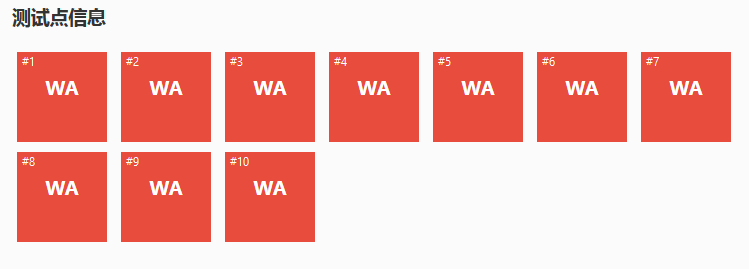//迟早会变成【真】的

2017 年 11 月 12 日，2017 年全国青少年信息学奥林匹克联赛 (NOIP2017)DAY2 交卷后。阴天。

DAY2 出来以后，大家貌似都比 DAY1 轻松，至少——我是这样的。

#include <bits/stdc++.h>
using namespace std;
template<typename _Tp>inline void IN(_Tp&dig)
{
char c;bool flag=0;dig=0;
while(c=getchar(),!isdigit(c))if(c=='-')flag=1;
while(isdigit(c))dig=dig*10+c-'0',c=getchar();
if(flag)dig=-dig;
}
int T,n,h,r;
double x,y,z;
bool book,hbok;
queue<int> que;
inline double P2(double a){return a*a;}
inline double dis(int a,int b)
{
return sqrt(P2(x[a]-x[b])+P2(y[a]-y[b])+P2(z[a]-z[b]));
}
int main()
{
freopen("cheese.in","r",stdin),freopen("cheese.out","w",stdout);
IN(T);
while(T--)
{
IN(n),IN(h),IN(r);
for(int i=1;i<=n;i++)IN(x[i]),IN(y[i]),IN(z[i]);
for(int i=1;i<=n;i++)
{
if(z[i]-r<=0)que.push(i),book[i]=1;
if(z[i]+r>=h)hbok[i]=1;
}
while(!que.empty())
{
int F=que.front();que.pop();
for(int i=1;i<=n;i++)if(!book[i])
if(dis(F,i)<=(double)(r<<1))
{
if(hbok[i]){puts("Yes");goto end;}
que.push(i),book[i]=1;
}
}
puts("No");
end:memset(book,0,sizeof(book));
memset(hbok,0,sizeof(hbok));
while(!que.empty())que.pop();
}
return 0;
}WTF？心头一紧，仔细检查，发现 freopen 关掉了啊。怎么回事？

#include <bits/stdc++.h>
using namespace std;
typedef long long LL;
template<typename _Tp>inline void IN(_Tp&dig)
{
char c;bool flag=0;dig=0;
while(c=getchar(),!isdigit(c))if(c=='-')flag=1;
while(isdigit(c))dig=dig*10+c-'0',c=getchar();
if(flag)dig=-dig;
}
int n,m,q,x,y;
map<int,LL> mp;
deque<LL> v1,v2;
void work()
{
for(int i=1;i<=m;i++)v1.push_back((LL)i);
for(int i=2;i<=n;i++)v2.push_back((LL)i*(LL)m);
for(int i=1;i<=q;i++)
{
LL tmp=v1[y[i]-1];
printf("%lld\n",tmp);
deque<LL>::iterator it=v1.begin()+y[i]-1;
v1.erase(it);
v2.push_back(tmp);
v1.push_back(v2),v2.pop_front();
}
}
LL getnum(int a,int b)
{
if(mp[a][b])return mp[a][b];
return (LL)(a-1)*(LL)m+(LL)b;
}
int main()
{
freopen("phalanx.in","r",stdin),freopen("phalanx.out","w",stdout);
IN(n),IN(m),IN(q);
int MX=1;
for(int i=1;i<=q;i++)IN(x[i]),IN(y[i]),MX=max(MX,x[i]);
if(MX==1)work(),exit(0);
for(int i=1;i<=q;i++)
{
LL tmp;
printf("%lld\n",tmp=getnum(x[i],y[i]));
for(int j=y[i];j<m;j++)mp[x[i]][j]=getnum(x[i],j+1);
for(int j=x[i];j<n;j++)mp[j][m]=getnum(j+1,m);
mp[n][m]=tmp;
}
return 0;
}


### 2 条评论#### fzj · 2017年11月13日 4:45 下午orz 我爆了好多分啊 药丸